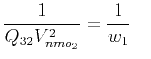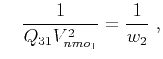On anelliptic approximations for qP velocities in TI and orthorhombic mediaNext: Examples Up: Proposed Approximations Previous: Proposed Approximations

### Moveout approximation

To convert the proposed group-velocity approximation (equation 47) to the corresponding moveout approximation, we apply again the general expression given in equation 31. Adopting the same notation rules, the moveout approximation takes the form:(54)where(55)(56)(57)denotes the offset indirection,denotes the offset indirection,denotes the NMO-velocity indirection,denotes the NMO-velocity indirection, anddenotes the hyperboloidal part of reflection traveltime squared given below,(58)

We apply the same strategy to reduce the number of parameters with an approximation onforas in equation 35.

For small offset, the Taylor expansion of equation 54 is(59)The asymptote of this expression for unbounded offsetsandis given byand(60)

which denote the horizontal velocities squared alonganddirections respectively.On anelliptic approximations for qP velocities in TI and orthorhombic mediaNext: Examples Up: Proposed Approximations Previous: Proposed Approximations

2017-04-14